• I was wondering if there is an equivalent way to add a row to a Series or DataFrame with a MultiIndex as there is with a single index, i.e. using .ix or .loc?I thought the natural way would be somethi...I was wondering if there is an equivalent way to add a row to a Series or DataFrame with a MultiIndex as there is with a single index, i.e. using .ix or .loc?
I thought the natural way would be something like
but that raises a KeyError. I know I can use .append(), but I would find it much neater to use .ix[] or .loc[].
here an example:
>>> df = pd.DataFrame({'Time': [dt.datetime(2013,2,3,9,0,1), dt.datetime(2013,2,3,9,0,1)], 'hsec': [1,25], 'vals': [45,46]})
>>> df
Time hsec vals
0 2013-02-03 09:00:01 1 45
1 2013-02-03 09:00:01 25 46
[2 rows x 3 columns]
>>> df.set_index(['Time','hsec'],inplace=True)
>>> ind = pd.MultiIndex.from_tuples([(dt.datetime(2013,2,3,9,0,2),0)],names=['Time','hsec'])
>>> df.ix[ind] = 5
Traceback (most recent call last):
File "", line 1, in
df.ix[ind] = 5
File "C:\Program Files\Python27\lib\site-packages\pandas\core\indexing.py", line 96, in __setitem__
indexer = self._convert_to_indexer(key, is_setter=True)
File "C:\Program Files\Python27\lib\site-packages\pandas\core\indexing.py", line 967, in _convert_to_indexer
raise KeyError('%s not in index' % objarr[mask])
KeyError: "[(Timestamp('2013-02-03 09:00:02', tz=None), 0L)] not in index"
解决方案
You have to specify a tuple for the multi-indexing to work (AND you have to fully specify all axes, e.g. the : is necessary)
In : df.ix[(dt.datetime(2013,2,3,9,0,2),0),:] = 5
In : df
Out:
vals
Time hsec
2013-02-03 09:00:01 1 45
25 46
2013-02-03 09:00:02 0 5
Easier to reindex and/or concat/append a new dataframe though. Generally setting (with this kind of enlargement), only makes sense if you are doing it with a small number of values. As this makes a copy when you do this.

展开全文• 1. 查看名称, 重命名.name方法, 查看Series的名称. .rename()方法, 重命名import 数据类型为字符串, 原Series名称不变2. 查看Series头部数据, 尾部数据.head()方法, 查看Series头部(前几个)数据. .tail()方法, 查看...

1. 查看名称, 重命名
.name方法, 查看Series的名称. .rename()方法, 重命名

import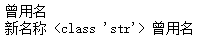数据类型为字符串, 原Series名称不变

2. 查看Series头部数据, 尾部数据

import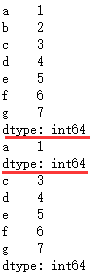3. Series数据索引 [超级重要]
3.0. 查看索引

import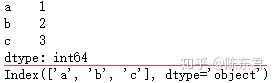3.1 标签索引

import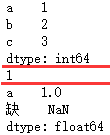如果index中不含索引的标签, 则返回NaN值

# 按属性,索引元素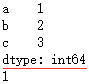3.2 下标索引
位置下标从0开始. 第一个元素下标索引为0

import pandas as pd
lst=[1,2,3]
s = pd.Series(lst,index=list("abc"))
print(s)
print(s[-2],type(s)) # 索引倒数第2个元素,并查看数据类型
print(s[[1,2]]) # 索引下标索引为1和2的元素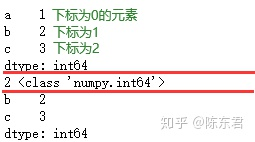3.3 切片索引

import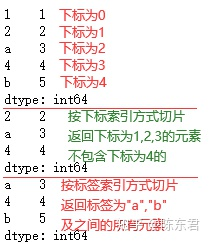切片索引与python中的list切片索引操作相同

import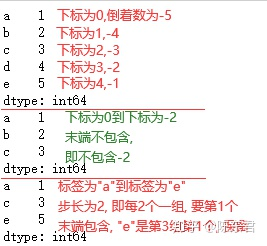3.4 布尔型索引
对Series进行判断, 可以生成布尔型的Series
布尔型索引, 保留生成的布尔型的Series中值为True的

import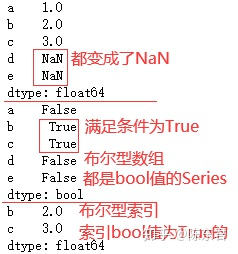.isnull()方法, 判断是否为空值

import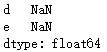.notnull()方法, 判断是否非空值, 用法同上
3.5. 重新索引

import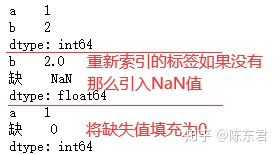4. Series排序
.sort_values()方法, 按值排序. .sort_index()方法, 按索引排序.

import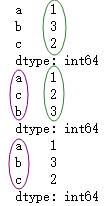4. Series对齐
Series之间的运算会按照index标签, 自动对齐, 与顺序无关.

import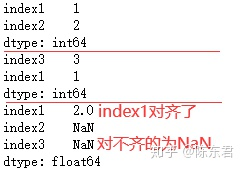5. 添加
5.1 标签索引添加

import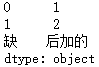5.2 .append(方法)添加

import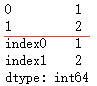6. 修改
标签索引修改

import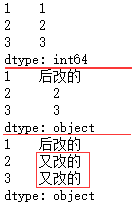7.删除
.drop()方法

import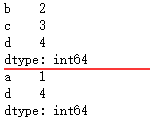详解
只索引单个元素时, 索引值必须包含在index中

import pandas as pd
lst=[1,2,3]
s = pd.Series(lst,index=list("abc"))
print(s)
print(s[["缺"]]) # index标签为"缺"的元素,组成的Series
print(s["缺"]) # index标签为"缺"的元素,由于不包含,所以报错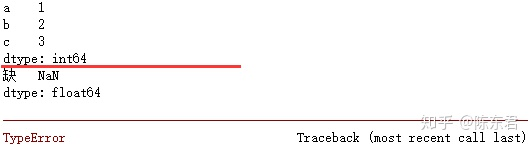得到Series, 由于不包含, 所以返回NaN值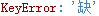部分报错内容, 大意就是index里没有*缺*这个key

纯数字的index, 不能用下标索引. python会认为是标签

import pandas as pd
lst=[1,2,3]
s = pd.Series(lst,index=[1,2,3])
print(s)
print(s) # 索引标签为1的元素,得到单个元素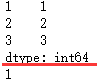展开全文• 使用Python数据分析，推荐安装Anaconda，建议使用Jupyter Notebook或Jupyter Lab。Python基础 Python3 基本数据类型 | 菜鸟教程Numpy导入import numpy as np创建np.array([1,2,3,4])切片dtype多维数组.T方法：转置，...

使用Python数据分析，推荐安装Anaconda，建议使用Jupyter Notebook或Jupyter Lab。
Python基础 Python3 基本数据类型 | 菜鸟教程
Numpy
导入
import numpy as np
创建
np.array([1,2,3,4])
切片
dtype
多维数组
.T方法：转置，例如原shape为(3,4)/(2,3,4)，转置结果为(4,3)/(4,3,2) → 所以一维数组转置后结果不变
numpy.reshape(a, newshape, order='C')：为数组提供新形状，而不更改其数据，所以元素数量需要一致！！
numpy.resize(a, new_shape)：返回具有指定形状的新数组，如有必要可重复填充所需数量的元素。
numpy.hstack(tup)：水平（按列顺序）堆叠数组
numpy.vstack(tup)：垂直（按列顺序）堆叠数组
numpy.stack(arrays, axis=0)：沿着新轴连接数组的序列，形状必须一样！
numpy.hsplit(ary, indices_or_sections)：将数组水平（逐列）拆分为多个子数组 → 按列拆分
numpy.vsplit(ary, indices_or_sections)：:将数组垂直（行方向）拆分为多个子数组 → 按行拆
print(ar.mean()) # 求平均值
print(ar.max()) # 求最大值
print(ar.min()) # 求最小值
print(ar.std()) # 求标准差
print(ar.var()) # 求方差
print(ar.sum(), np.sum(ar,axis = 0)) # 求和，np.sum() → axis为0，按列求和；axis为1，按行求和
print(np.sort(np.array([1,4,3,2,5,6]))) # 排序
numpy.random.rand(d0, d1, ..., dn)：生成一个[0,1)之间的随机浮点数或N维浮点数组 —— 均匀分布
numpy.random.randn(d0, d1, ..., dn)：生成一个浮点数或N维浮点数组 —— 正态分布
numpy.random.randint(low, high=None, size=None, dtype='l')：生成一个整数或N维整数数组
存储数组数据 .npy文件
np.save() #保存
Pandas
导入
import pandas as pd
创建
pd.Series([1,2,3,4])
pd.DataFrame(np.random.rand(10,5))
数据导入
……
数据导出
df.to_csv(filename)
……
数据查看
df.tail(n) # 查看数据框的最后n行
df.shape # 查看数据框的行数与列数
df.info() # 查看数据框 (DataFrame) 的索引、数据类型及内存信息
df.describe() # 对于数据类型为数值型的列，查询其描述性统计的内容
s.value_counts(dropna=False) # 查询每个独特数据值出现次数统计
df.apply(pd.Series.value_counts) # 查询数据框 (Data Frame) 中每个列的独特数据值出现次数统计
数据选取
df[col] # 以数组 Series 的形式返回选取的列
df[[col1, col2]] # 以新的数据框(DataFrame)的形式返回选取的列
s.iloc # 按照位置选取
s.loc['index_one'] # 按照索引选取
df.iloc[0,:] # 选取第一行
df.iloc[0,0] # 选取第一行的第一个元素
数据清洗
df.columns = ['a','b'] # 重命名数据框的列名称
pd.isnull() # 检查数据中空值出现的情况，并返回一个由布尔值(True,Fale)组成的列
pd.notnull() # 检查数据中非空值出现的情况，并返回一个由布尔值(True,False)组成的列
df.dropna() # 移除数据框 DataFrame 中包含空值的行
df.dropna(axis=1) # 移除数据框 DataFrame 中包含空值的列
df.fillna(x) # 将数据框 DataFrame 中的所有空值替换为 x
s.fillna(s.mean()) -> 将所有空值替换为平均值
s.astype(float) # 将数组(Series)的格式转化为浮点数
s.replace(1,'one') # 将数组(Series)中的所有1替换为'one'
s.replace([1,3],['one','three']) # 将数组(Series)中所有的1替换为'one', 所有的3替换为'three'
df.rename(columns=lambda x: x + 2) # 将全体列重命名
df.rename(columns={'old_name': 'new_ name'}) # 将选择的列重命名
df.set_index('column_one') # 改变索引
df.rename(index = lambda x: x+ 1) # 改变全体索引
df[df[col] > 0.5] # 选取数据框df中对应行的数值大于0.5的全部列
df[(df[col] > 0.5) & (df[col] < 0.7)] # 选取数据框df中对应行的数值大于0.5，并且小于0.7的全部列
df.sort_values(col1) # 按照数据框的列col1升序(ascending)的方式对数据框df做排序
df.sort_values(col2,ascending=False) # 按照数据框的列col2降序(descending)的方式对数据框df做排序
df.sort_values([col1,col2],ascending=[True,False]) # 按照数据框的列col1升序，col2降序的方式对数据框df做排序
df.groupby(col) # 按照某列对数据框df做分组
df.groupby([col1,col2]) # 按照列col1和col2对数据框df做分组
df.groupby(col1)[col2].mean() # 按照列col1对数据框df做分组处理后，返回对应的col2的平均值
df.pivot_table(index=col1,values=[col2,col3],aggfunc=mean) # 做透视表，索引为col1,针对的数值列为col2和col3，分组函数为平均值
df.groupby(col1).agg(np.mean)
df.apply(np.mean) # 对数据框df的每一列求平均值
df.apply(np.max,axis=1) # 对数据框df的每一行求最大值
数据连接
df1.append(df2) # 在数据框df2的末尾添加数据框df1，其中df1和df2的列数应该相等
pd.concat([df1, df2],axis=1) # 在数据框df1的列最后添加数据框df2,其中df1和df2的行数应该相等
df1.join(df2,on=col1,how='inner') # 对数据框df1和df2做内连接，其中连接的列为col1
数据统计
df.describe() # 得到数据框df每一列的描述性统计
df.mean() # 得到数据框df中每一列的平均值
df.corr() # 得到数据框df中每一列与其他列的相关系数
df.count() # 得到数据框df中每一列的非空值个数
df.max() # 得到数据框df中每一列的最大值
df.min() # 得到数据框df中每一列的最小值
df.median() # 得到数据框df中每一列的中位数
df.std() # 得到数据框df中每一列的标准差
Python连接数据库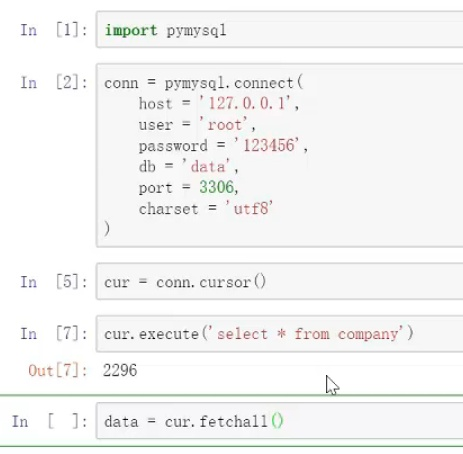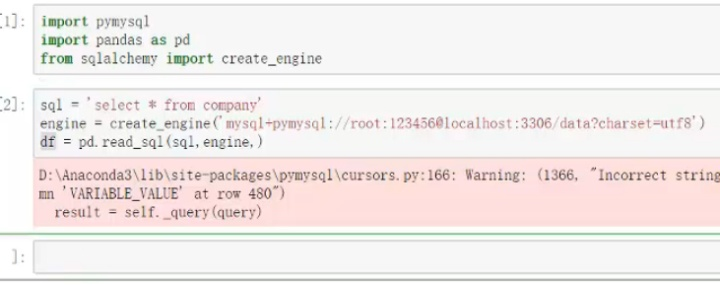可视化
matplotlib 的数据可视化

展开全文• 、介绍pandas 是基于NumPy的种工具，该工具是为了解决数据分析任务而创建的。Pandas 纳入了大量库和一些标准的数据模型，提供了高效地操作...2. pandas中提供了两种常见的数据类型：Series二维 DataFram...一、介绍
pandas 是基于NumPy的一种工具，该工具是为了解决数据分析任务而创建的。Pandas 纳入了大量库和一些标准的数据模型，提供了高效地操作大型数据集所需的工具。pandas提供了大量能使我们快速便捷地处理数据的函数和方法。你很快就会发现，它是使Python成为强大而高效的数据分析环境的重要因素之一。
2. pandas中提供了两种常见的数据类型：
一维 Series二维 DataFrame
二、使用及安装方式
安装

# 方法一：


导入，pandas习惯被简写成pd

import pandas as pd

三、Series类型
Series类型类似于Numpy的一维数组对象，可以将该类型看做是一组数据与数据相关的标签（索引）联合而构成（带有标签的一维数组对象）。
创建方式
列表

s=pd.Series([1,2,3],index=list("abc"))

字典

s=pd.Series({"a":1,"b":2,"c":3})

numpy中的ndarray数组

s=pd.Series(np.array([1,2,3]),index=list("abc"))

标量创建

s=pd.Series(1,index=list("abc"))

2. 相关属性
index 索引values 值shape 形状size 元素个数dtype 类型head/tail 首/尾元素
3. 相关操作
矢量化运算
运算符

s1.add(s2,fill_value=1)

isnull/notnull 空值判断统计函数sum、min、max、min...，series在运算nan时会忽略nan，比如求和时结果部位nan
4. 索引和切片
如果Series对象的index值为非数值类型，通过[索引]访问元素，索引既可以是标签索引，也可以是位置索引。这会在一定程度上造成混淆。我们可以通过：
loc 仅通过标签索引访问，包左包右。iloc 仅通过位置索引访问，包左不包右。
这样，就可以更加具有针对性去访问元素。
也可以通过布尔值数组作为索引。
5. CURD
获取和修改 loc、iloc追加
方法一：loc[索引]=值
方法二：
reindex(新索引,method='填充方式',limit=填充次数)
删除 drop('索引',inplace=TrueFalse)
四、DataFrame类型
DataFrame是一个多维数据类型。因为通常使用二维数据，因此，我们可以将DataFrame理解成类似excel的表格型数据，由多列组成，每个列的类型可以不同。
因为DataFrame是多维数据类型，因此，DataFrame既有行索引，也有列索引。
创建方式
数组

df=pd.DataFrame([[1,2,3],[4,5,6]],index=["A","B"],columes=["a","b","c"])

字典

pd

2. 相关属性
index行索引columns 列索引values 值shape 形状ndim 维度dtypes 类型
3. 相关操作
列操作
获取列

df[列索引]
df.列索引

增加修改列

df[列索引]=列数据

删除列

del df[列索引]

# pop 删除指定索引的元素，返回被删除的元素，只能删除一列
df.pop(列索引)

# axis在dataframe中，不一定指定0就是垂直方向，1就是水平方向。
df.drop(列索引或数组,axis=10)

行操作
获取行

df.loc[行索引]
df.iloc[行编号]
df[[布尔值]]

增加行

#追加单行
df.append(row)

#追加多行，append也可以，但是不适合
pd.concat((df1,df2),ignore_index=True)

行列混合操作
切片

# 先获取行
df.loc[].loc[]

# 先获取列
df[].loc[]

# 混合切片
# 切片只能从高维切到低维。
# 所以，对于dataframe行列的混合切片，只能先切行，再切列
# （1）loc
df.loc[标签start:标签end:step,低维切片(按照标签)]  # 包含end
#  (2)iloc
df.iloc[位置start:位置end:step,低维切片（按照位置）]  # 不包含end

混合运算
DataFrame的一行或一列都是Series类型的对象。因此，DataFrame可以近似看做是多行或多列Series构成的，Series对象支持的很多操作，对于DataFrame对象也同样适用，我们可以参考之前Series对象的操作。
转置：
a. DataFrame进行运算时，会根据行索引与列索引进行对齐。当索引无法匹配时，产生空值（NaN）。如果不想产生空值，可以使用DataFrame提供的运算函数来代替运算符计算，通过fill_value参数来指定填充值。
b. DataFrame与Series混合运算。【默认Series索引匹配DataFrame的列索引，然后进行行广播。可以通过DataFrame对象的运算方法的axis参数，指定匹配方式（匹配行索引还是列索引）。

df1+df2

# 【注意】填充值只能够当其中一个dataframe有效，如果两个dataframe的值都是nan
df1.add(df2,fill_value=0)

行列转换

df1.T

a. 索引排序
Series与DataFrame对象可以使用sort_index方法对索引进行排序。DataFrame对象在排序时，还可以通过axis参数来指定轴（行索引还是列索引）。也可以通过ascending参数指定升序还是降序。

df.sort_index(axis=0,ascending=False)

b. 值排序
Series与DataFrame对象可以使用sort_values方法对值进行排序。

# axis
# by：按照哪个索引进行排序
#     排的是行，按照哪个列索引进行排序
#     排的是列，按照哪个行索引进行排序
# inplace
# asending ：默认True 升序
df.sort_values(axis= ,by= )

c. 排名方法

#参数
# method：默认并列元素 ，显示均值
#         也可以设置max，min ，first（按照数据在原始数据中出现的顺序排名）
# s.rank(method="min")

统计方法
mean / sum / count
max / min
cumsum / cumprod 累加/累乘
idxmax / idxmin
var / std 方差/标准差
corr / cov 相关系数/协方差
其他
unique

unique 只能去除重复元素，不能排序（ndarray数组是可以排序的）

value_counts

value_counts返回series中每个值出现的次数，默认按照降序排列

isin

isin 可以使用与serise，也可以适用于dataframe，判断参数是否存在于pandas对象中；
也可以判断原元素的交集 True的是交集的部分。


展开全文• python操作数据库# coding: utf-8 二 pandas清洗数据一览数据面貌df获取查询数据末尾5pd.value_counts(Series)统计Series中不同元素出现的次数data各个年级出现的次数reset_index将serives转化为dataframedata=...
• 数据是可视化展示的实体，因此要会利用现成Python库进行数据读取和后续处理操作。记录学习路上的点滴，周，月，一年...总会有收获的，不当工作，只为兴趣。写在开始之前 本文和后续文章用到的数据是从 UCI公开...
• 问题： 现在手中有表格，其中列数据长成下列格式：三个元素挤在个单元格中，现在需要把这三个元素进行分隔开，生成新的三列，该如何办？前面介绍了str.split()方法和re.split()方法，利用这两个方法任意种...
• 需求：写个汇总 分析：concat, merge, df.loc[xx] = [a,b,c] 都可以满足 也可像 list 一样用append 1.append(series) data = pd.DataFrame() series = pd.Series({"x":1,"y":2},name="a") data = data.append...
• 内容目录Series简介Series的创建方式Series索引和切片Series基本操作1...pandas的两大主要数据结构 Series和DateFrame，其中Series 是带标签的维数组，可存储整数、浮点数、字符串、Python 对象等类型的数据。轴标...
• Python numpy 提取矩阵的某一行或某一列的实例如下所示：import numpy as npa=np.arange(9).reshape(3,3)aOut:array([[0, 1, 2],[3, 4, 5],[6, 7, 8]])矩阵的某一行aOut: array([3, 4, 5])矩阵的某一列a...
• python中对已经生成的Series，怎样组合成DataFrame如 a = Series([1,2,3]) b = Series([2,3,4]) 怎样将a b组合成一个DataFzip函数接受任意多个（包括0个和1个）序列作为参数，返回个tuple列表。具体意思不好用文字...
• 今天粉丝提了下面这样一个问题，其中一个是”一行拆多行”，另外一个是”多行并一行”，貌似群友用power query已经解决了。但是基于Python怎么做呢？接着往下看。 一行拆多行 上面这个问题我会提供两个思路，供...
• Series: 维数据对象     今天简单介绍一下series这种数据对象。     Series种类似于维数组的对象，由组数据和组与之相关的数据标签（索引)组成，Series比较像...数据分析 缺失值处理
• 讲我们讲到了Python 针对Excel 里面的特殊数据处理以及各种数据统计，本讲我们将引入Pandas 这个第三方库来实现数据的统计，只要个方法就可以统计到上讲的数据统计内容，本讲也会扩展讲讲Pandas所涉及到的...
• I have a Python dataFrame with multiple columns.LogBlk Page BayFail0 0 [0, 1, 8, 9]1 16 [0, 1, 4, 5, 6...
• ## python 创建Series

千次阅读 2019-09-22 22:05:02
#用于存储一行或一列的数据,以及与之相关的索引的集合 import pandas as pd s1 = pd.Series([43,56]) print(s1) s2 = pd.Series([43,12.4]) print(s2) s3 = pd.Series([False,True]) print(s3) #有一个是对象，...
• Python中实现"一行拆多行"和"多行并一行"，你会吗？
• 请关注支持下作者的公众号：极客挖掘机，您的关注，是对小编坚持原创的最大鼓励：)人生苦短，我选Python在这里首先恭喜各位看到本篇连载的同学，本篇连载为 《小白学 Python 基础系列》 最后篇，恭喜各位在学习 ...
• 】●series相加我们知道两个字符串相加就会拼接成个新的字符串，那么pandas中dataframe的两个series相加是什么结果呢？如果这个dataframe的具体的某个数据的dtype是int和float类型，相加的效果就相当于两个数...
• series和dataframe进行groupby操作以后的对象总是多了一行索引，以前就遇到过这个问题i，一直没弄明白，原来是一个参数没弄对 df.groupby(by=‘A’, group_keys=False) 只要加group_keys=False这个参数就可以了，...
• 如题：python如何删除一列 python如何删除一行 list列表如何删除任意一行或一列 numpy如何删除一行或一列 利用numpy可以删除list的行或者列 删除一行 import numpy as np list_data = [[1,2,3],[2,3,4],[4,5,6],[9,8...
• from  ... 、reindex() 方法：重新索引 ...针对 Series ...如果传入的索引值在数据里不存在，则不会报错，而是添加缺失值的新。 不想用缺失值，可以用 fi
• Let's say that I have a dataframe like this oneimport pandas as pddf = pd.DataFrame([[1, 2, 1], [1, 3, 2], [4, 6, 3], [4, 3, 4], [5, 4, 5]], columns=['A', 'B', 'C'])>> dfA B C0 1 2 11 1 3...
• # dataframe的每一列除以series，如果想要每一行除以series，则可采用转置T data = {'hah':[1,2,2], '数量':[3,2,5], '价格':[10,9,8]} df = pd.DataFrame(data) print(df) from pandas import Series, DataFrame...
• 今天小编就为大家分享python 创建个空dataframe 然后添加行数据的实例，具有很好的参考价值，希望对大家有所帮助。一起跟随小编过来看看吧
• 这里假设已经能够熟练使用Pandas库了，获得Series，需要获得第个值，而且第个值对应大索引值不为0的情况下面使用。 解决 df['A'].iloc 参考 Get first element of Series without knowing the ...
• Python之Pandas中Series、DataFrame实践1. pandas的数据结构Series1.1 Series种类似于维数组的对象，它由组数据（各种NumPy数据类型）以及组与之相关的数据标签（即索引）组成。 1.2 Series的字符串表现...pandas dataframe
• #忽略索引,往dataframe中插入一行数据 # print(df) i = i + 1 # print(dframe1) # print(sku.values) #将series变成数组 print(df) df = df[["sku","ebayno","sale"]] #选取指定的列 df.to_csv("std.csv",encoding=...
• ## Python之Series 学习

千次阅读 2017-02-06 09:04:28
pandas 是基于 Numpy 构建的含有更高级数据结构和工具的数据分析包 ...Series 和 DataFrame 分别对应于维的序列和二维的表结构。pandas 约定俗成的导入方法如下：from pandas import Series,DataFrame i...

# pythonseries增加一行python 订阅